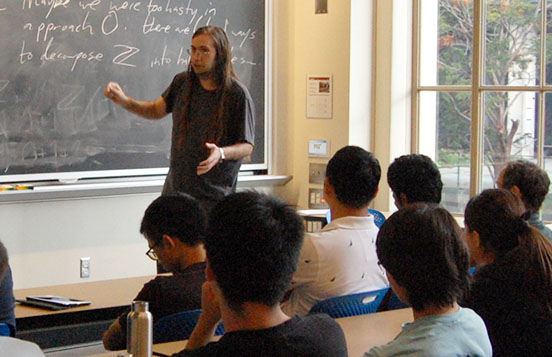# RSI/SPUR Lecture SeriesAaron Pixton's talk in the 2018 RSI/SPUR Lecture Series

This lecture series is organized by SPUR/RSI faculty advisors Davesh Maulik and Ankur Moitra. These summer lectures are aimed at our RSI and SPUR students.

• July 1, Peter Hintz

Scattering theory and resonances

What do you hear when you strike a bell or pluck a guitar string? A note which has a certain frequency and fades out at a certain rate. (If you listen carefully, you hear a superposition of such notes.) Scattering theory is the study of "open systems", such as these two, in which energy can escape from bounded regions off to infinity; resonances describe the characteristic frequencies (and decay rates) of oscillation of open systems. I will explain what this means in mathematical terms and present examples ranging from toy models to dynamical black holes and the gravitational waves emitted by them.

• July 8, Nike Sun

Sharp transitions in computational problems

I will talk about randomized computational problems, such as the random k-SAT problem, or the maximum independent set problem on random graphs. Many of these problems exhibit "phase transition" phenomena, where the behavior of the system changes drastically under a very small change in the model parameters. I will survey some of the progress on understanding these phenomena, which has brought together ideas from statistical physics, computer science, combinatorics, and probability.

• July 15, Bjorn Poonen

The abc conjecture

The abc conjecture is an innocent-looking statement about integer solutions to the equation a+b=c that would have profound implications in number theory. I will explain what it says, why it is interesting, and why there is controversy about whether it has been proved.

• July 22, Pavel Etingof

Iterating sine, equivalence classes of variable changes, and finite groups with few conjugacy classes

I will talk about the following three problems:

1. What happens if you repeatedly press the "sine" button on your calculator?
2. What are equivalence classes of variable changes y=f(x) where f(0)=0 and f'(0)=1?
3. How to construct "the most noncommutative" finite groups, i.e. ones with as few conjugacy classes as possible?

Are you wondering what these problems are doing in the same talk? Come and find out!